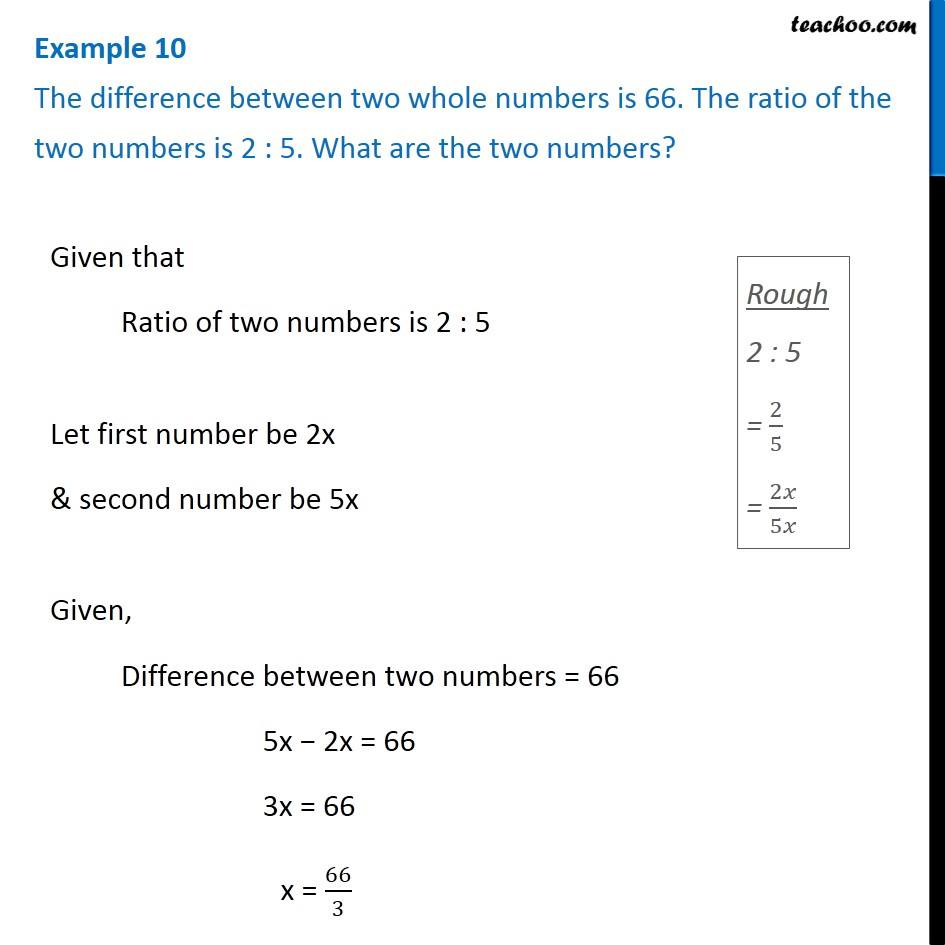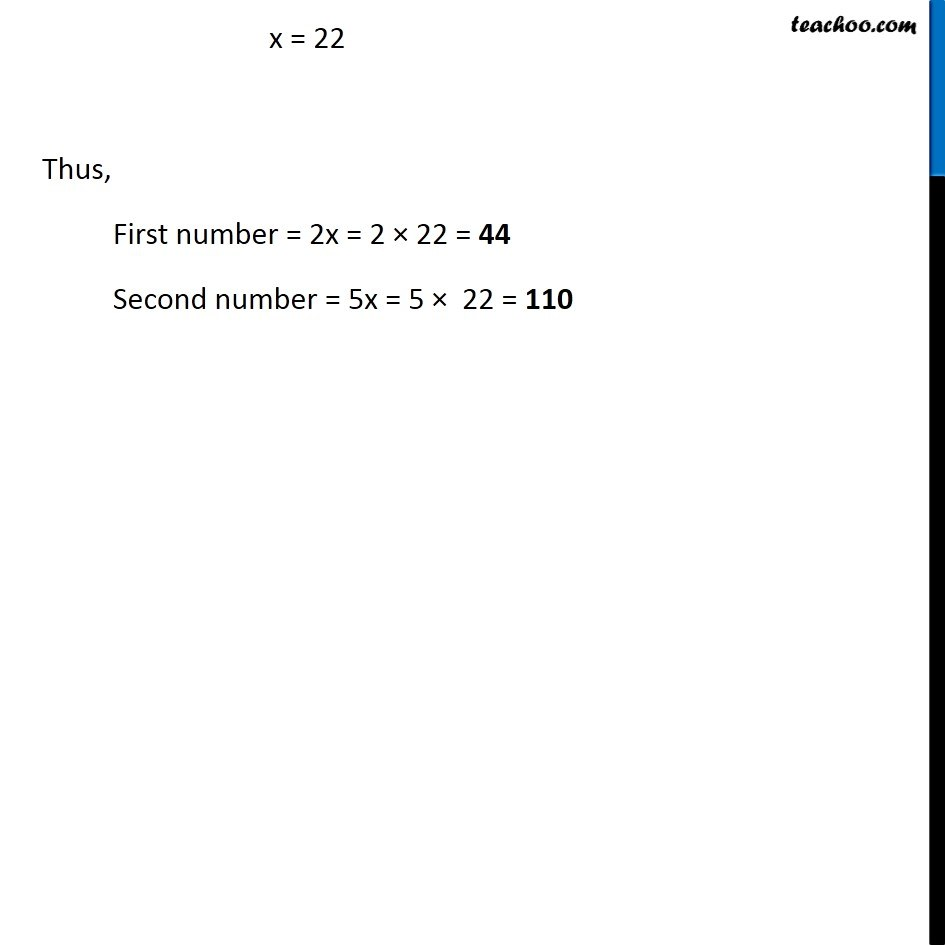1. Chapter 2 Class 8 Linear Equations in One Variable
2. Concept wise
3. Forming Linear Equations - Adding subtracting numbers

Transcript

Example 10 The difference between two whole numbers is 66. The ratio of the two numbers is 2 : 5. What are the two numbers?Given that Ratio of two numbers is 2 : 5 Let first number be 2x & second number be 5x Given, Difference between two numbers = 66 5x − 2x = 66 3x = 66 x = 66/3 x = 22 Thus, First number = 2x = 2 × 22 = 44 Second number = 5x = 5 × 22 = 110

Forming Linear Equations - Adding subtracting numbers